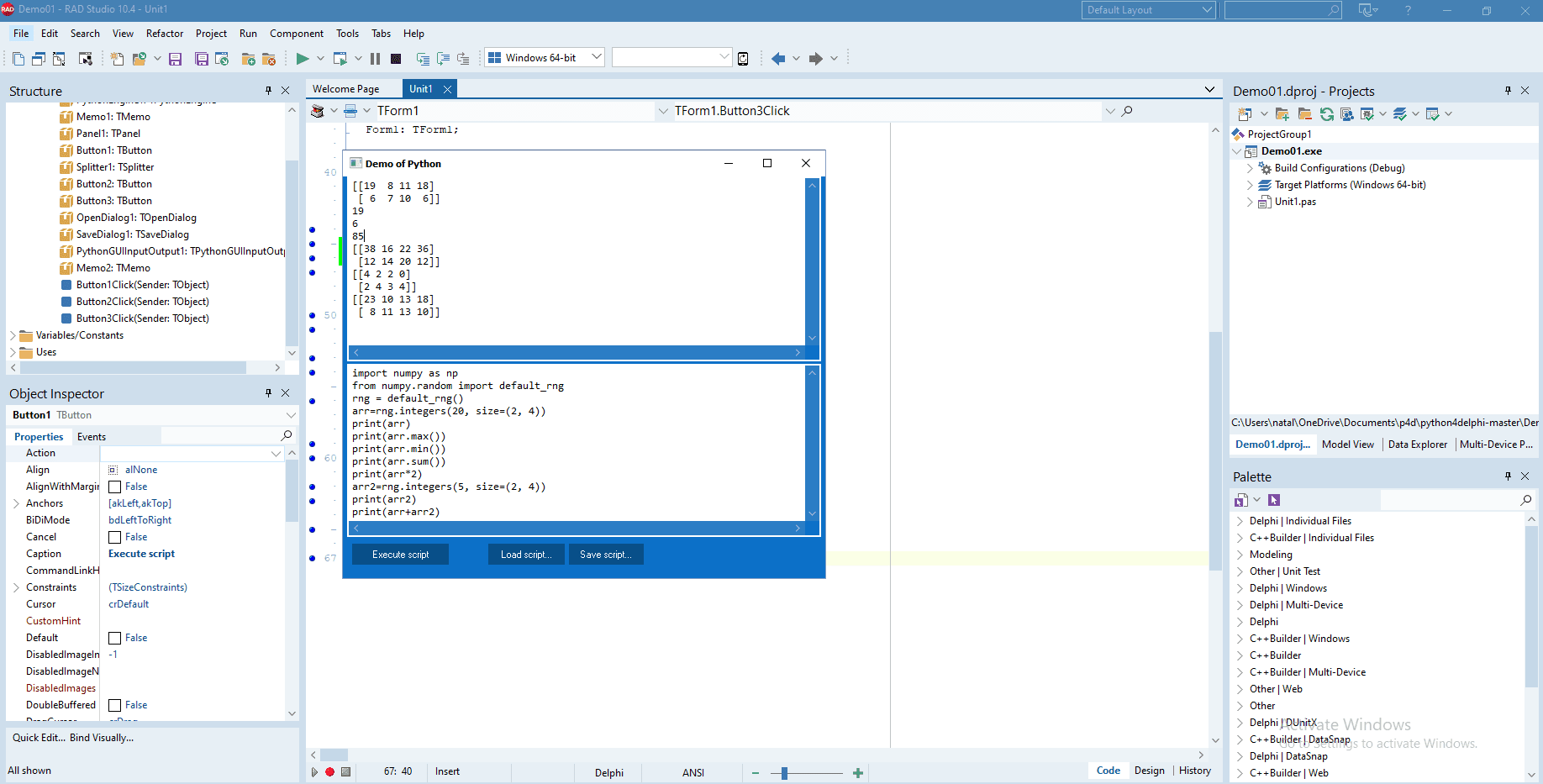# Build A Numpy Library Python GUI In A Delphi Windows GUI AppIf you need to perform complex transformations or mathematical calculations with matrices or arrays, then Python Numpy library is exactly what you need. You can easy run this library with Python4Delphi (P4D). Python4Delphi is a free tool with which you can work with Python scripts and objects in the Windows GUI. In this post, we will look at how to run Numpy library with P4D.

Now you can build Python GUI apps for Windows using a lot of Python libraries with Delphi and C++Builder and Python4Delphi. Just open and run Demo1 project. Then paste the Python script into the text field, press Execute button and get the result. Go to GitHub to download Demo1.

Numpy library allows you to create multidimensional arrays and matrices and work with their properties. It also contains various functions for processing arrays and matrices. Let’s look at some simple examples of working with Numpy. If you have trouble compiling the Python you may need to have Numpy 1.19.3 installed.

### Create a matrix and get some properties

This example shows how to create a 3-dimensional array and fill it with numbers from 0 to 29. Then, using the properties of this array, we can find out its shape, dimension, data type, number of elements.

### Basic operations with arrays

Let’s take a look at the simplest conversions you can perform on arrays. Using concatenate() function, you can combine the values of two arrays into one. With function sort() you can sort ascending the values in an array. Function reshape() allows you to change the dimension of the array.

### Mathematical operations with matrix

Function default_rng()  allows you to fill a matrix with random values. You can use integer or real numbers. In this example, we fill the matrix with integer values. Then we find the maximum and minimum element, the sum of all the elements in the matrix. It is also shown how you can multiply a matrix by a number and sum two matrices with the same dimension.And this is only a small part of what Numpy library allows you to do. Download Numpy and check how many possibilities it opens for your applications. Install Python4Delphi for building Python GUIs for Windows using Delphi easily.

### Watch the Python GUI Apps Con 2023 sessions today!